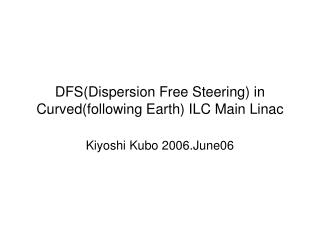Download PresentationDFS(Dispersion Free Steering) in Curved(following Earth) ILC Main Linac# DFS(Dispersion Free Steering) in Curved(following Earth) ILC Main Linac - PowerPoint PPT Presentation

Download Presentation##### DFS(Dispersion Free Steering) in Curved(following Earth) ILC Main Linac

Download Policy: Content on the Website is provided to you AS IS for your information and personal use and may not be sold / licensed / shared on other websites without getting consent from its author. While downloading, if for some reason you are not able to download a presentation, the publisher may have deleted the file from their server.

- - - - - - - - - - - - - - - - - - - - - - - - - - - E N D - - - - - - - - - - - - - - - - - - - - - - - - - - -
##### Presentation Transcript

1. DFS(Dispersion Free Steering) in Curved(following Earth) ILC Main Linac Kiyoshi Kubo 2006.June06

2. Lattice • For Performance Study by P. Tenenbaum • one quad per four cryomodules • 75/65 degree phase advance per FODO cell

3. Curved Linac, 1-quad/4-cryomodules Alignment line BPM-Quad-Dipole package Beam orbit BPM-Quad-Dipole package BPM (zero length) Dipole corrector (zero length) Quad magnet (0.66 m)

4. Design orbit and dispersion

5. Emmitance without errors

6. Definition of Projected emittance and Linear Dispersion Corrected emittance

7. Simulated Algorithm of DFS, mode 0 One-to-one orbit correction (BPM reading zeroed) Divide linac into sections (can be overlapped) and in each section: (1) Measure orbit with nominal beam energy. (y0,i at i-th BPM) (2) Reduce initial beam energy and accelerating gradient in entire linac by a common factor  (e.g. 10% or = -0.1). (3) For the second section or downstream, orbit adjusted at the two BPMs just before the section to make the position at the BPM y = y0 +  (y0 is the position with nominal energy,  the dispersion at BPM.) (4) Measure orbit. (y,i at i-th BPM) (5) Set dipole correctors in the section to minimize w(y,i - y0,i - i)2 + y0,i - ydes,i)2 (i is the dispersion, ydes,i the designed orbit at i-th BPM. w is the weight factor, chosen as w=5000.). (6) Iterate from (1) to (5). (7) Go to next section.

8. Simulated Algorithm of DFS, mode 1 One-to-one orbit correction (BPM reading zeroed) Divide linac into sections (can be overlapped) and in each section: (1) Measure orbit with nominal beam energy. (y0,i at i-th BPM) (2) Reduce initial beam energy and accelerating gradient from the linac entrance to the entrance of the section by a common factor  (e.g. 10% or = -0.1). (3) For the second section or downstream, orbit adjusted at the two BPMs just before the section to make the position at the BPM y = y0 +  (y0 is the position with nominal energy,  the dispersion at BPM.) (4) Measure orbit. (y,i at i-th BPM) (5) Set dipole correctors in the section to minimize w(y,i - y0,i - ycal,i)2 + y0,i - ycal,i)2 (ycal,i is the calculated orbit difference, ycal,I the calculated orbit, without errors, at I-th BPM. w is the weight factor, w=5000.). (6) Iterate from (1) to (5). (7) Go to next section.

9. Simulated Algorithm of DFS, mode 2 One-to-one orbit correction (BPM reading zeroed) Divide linac into sections (can be overlapped) and in each section: (1) Measure orbit with nominal beam energy. (y0,i at i-th BPM) (2) Reduce initial beam energy and accelerating gradient from the linac entrance to the entrance of the section by a common factor  (e.g. 10% or = -0.1). (3) (No upstream orbit adjustment) (4) Measure orbit. (y,i at i-th BPM) (5) Set dipole correctors in the section to minimize w(y,i - y0,i - ycal,i)2 + y0,i - ycal,i)2 (ycal,i is the calculated orbit difference, ycal,I the calculated orbit, without errors, at I-th BPM. w is the weight factor, w=5000.). (6) Iterate from (1) to (5). (7) Go to next section.

10. Example of beam orbit after DFS Quad offset 0.3 mm, Cavity offset 0.5 mm, Quad-BPM offset 0.2 mm, Cavity tilt 0.5 mrad, BPM resolution 3 micron. (example of one particular seed.)

11. Sensitivity of final (linear dispersion corrected) emittance to various errors(Average of 25 random seeds. No other errors in each figure.)

12. Emittance vs. s Quad offset 0.3 mm, Cavity offset 0.5 mm, Quad-BPM offset 0.2 mm, Cavity tilt 0.5 mrad, BPM resolution 3 micron. (Average of 100 random seeds.) Mode 1 shows clear structure due to division into sections.

13. Summary and comments • DFS (Dispersion Free Steering) is effective in slowly curved (following earth) linac. • Three different algorithms of DFS were tested. • Result of mode 0 is most sensitive to cavity tilt error, as expected. • Averaged emittance of the three looked similar for the combined errors: Quad offset 0.3 mm, Cavity offset 0.5 mm, Quad-BPM offset 0.2 mm, Cavity tilt 0.5 mrad, BPM resolution 3 micron. • About 40% to 50% vertical emittance dilution. (w.r.t. 2E-8 m) • The results seem to depend on how the linac is divided into sections. (not shown here)